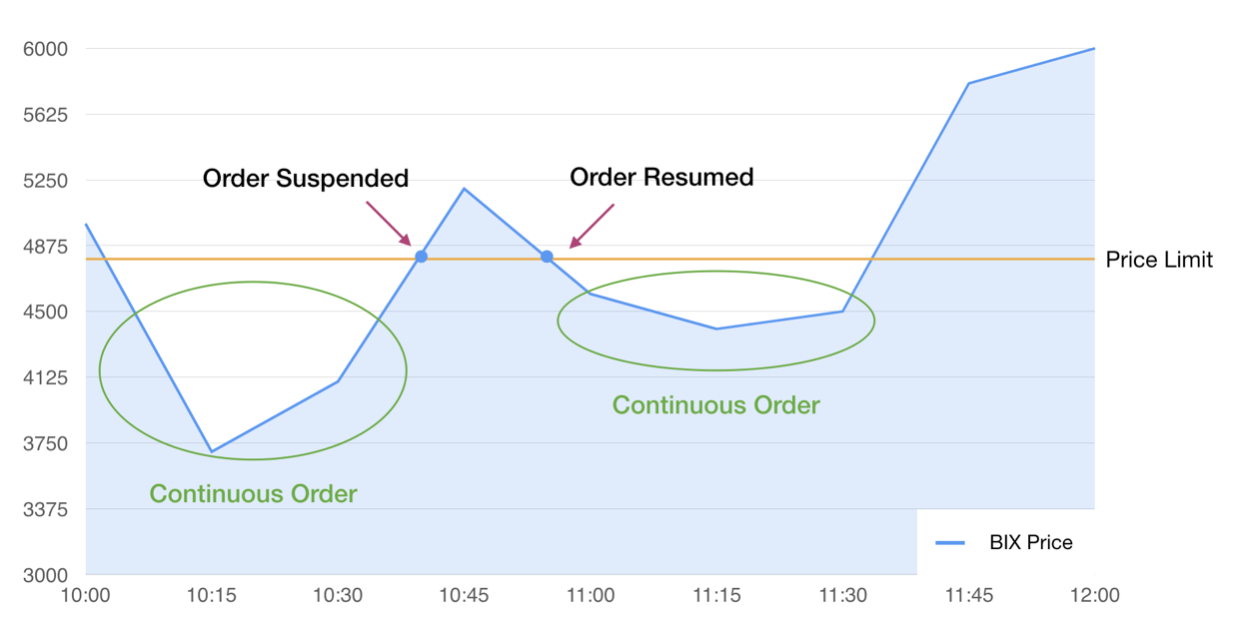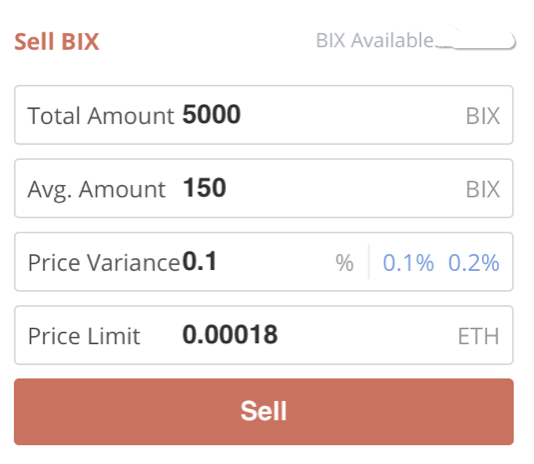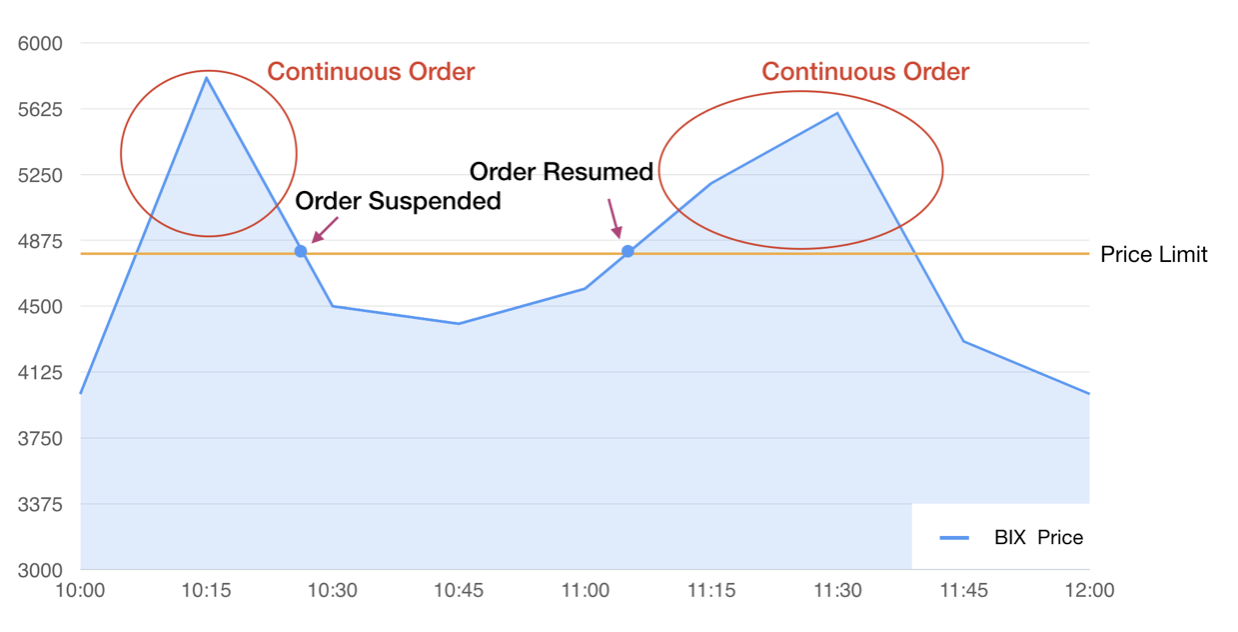Back
Iceberg Order Instructions

Split a large Buy/Sell order into several small orders at order prices adjusted based on real-time price fluctuations to reduce the impact on the market price.

Investor A can set an "Iceberg Order" if he intends to buy an amount of BIX equivalent to 100 ETH with minimal impact on the market price.

Set the order to buy 100 ETH and the average amount of each split order to be 0.1 ETH (The actual order amount would fluctuate between 90% and 110% of the average amount). You may also adjust the price variance and set the maximum purchase price.Click on “Buy” and the system will implement the Iceberg Order for Investor A:

- The total order amount will be the "Total Amount" set by Investor A

- Each Order Amount of the Iceberg Order will be 90%-110% Of Avg. Amount set by Investor A

- The Order Price will be "Price * (1 - Price Variance)"

- And a new order will be created after the previous one has been made under the above rules

- The order will be automatically cancelled and re-ordered if the difference between the "real-time price" and the order price exceeds "The real-time price * (1 - Price Variance*2)"

- The order will be suspended if the "real-time price" exceeds the "price limit" set by Investor A and will resume when the real-time price is lower than the price limit.

- The order will be automatically suspended when the "Total Trade Volume" of the order reaches the "Order Amount".--------------------------------------------------------------------------------------------------------------

Case 2：Iceberg Sell Order

Investor A can set an "Iceberg Order" if he intends to sell 5,000 BIX without affecting the market to prevent decrease in his revenue in the case of price decrease.

Set the order to sell 5,000 BIX and the average amount of each split order to be 150 BIX (The actual order amount would fluctuate between 90% and 110% of the average amount). You may also adjust the price variance and set the minimum sell price.Click on “Sell” and the system will implement the Iceberg Order for Investor A:

- The total order amount will be the "Total amount" set by Investor A

- Each Order Amount of the Iceberg Order will be 90%-110% Of Avg. Amount set by Investor A

- The Order Price will be "Price * (1 + Price Variance)"

- And a new order will be created after the previous one has been made under the above rules

- The order will be automatically cancelled and re-ordered if the difference between the "real-time price" and the order price exceeds "The real-time price * (1 + Price Variance*2)"

- The order will be suspended if the "real-time price" is lower than the "price limit" set by Investor A and will resume when the real-time price is higher than price limit.

- The order will be automatically suspended when the "Total Trade Volume" of the order reaches the "Order Amount".